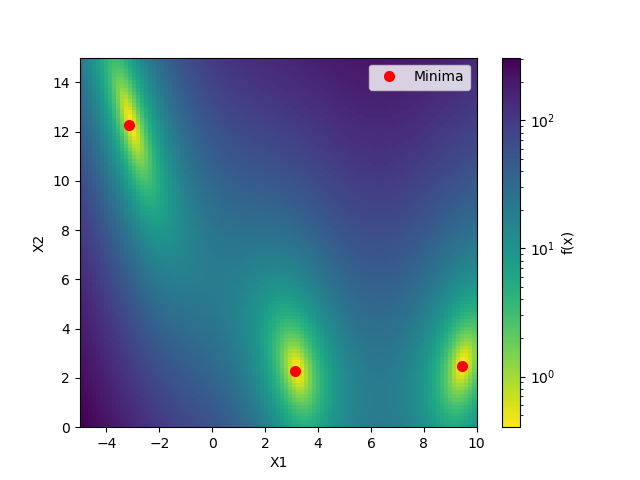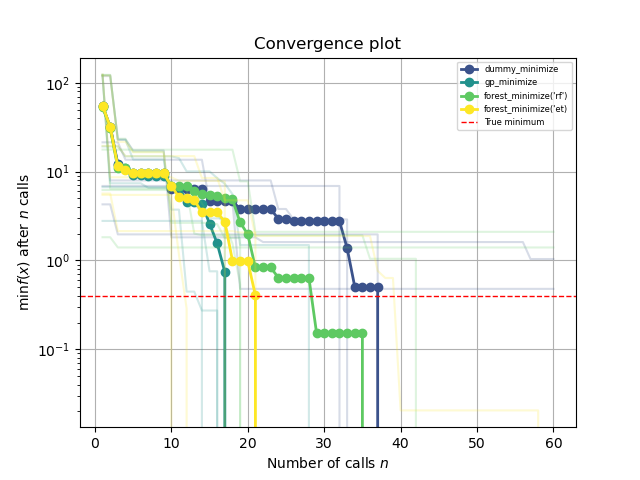# Comparing surrogate models¶

Tim Head, July 2016. Reformatted by Holger Nahrstaedt 2020

Bayesian optimization or sequential model-based optimization uses a surrogate model to model the expensive to evaluate function func. There are several choices for what kind of surrogate model to use. This notebook compares the performance of:

• gaussian processes,

• extra trees, and

• random forests

as surrogate models. A purely random optimization strategy is also used as a baseline.

print(__doc__)
import numpy as np
np.random.seed(123)
import matplotlib.pyplot as plt


## Toy model¶

We will use the benchmarks.branin function as toy model for the expensive function. In a real world application this function would be unknown and expensive to evaluate.

from skopt.benchmarks import branin as _branin

def branin(x, noise_level=0.):
return _branin(x) + noise_level * np.random.randn()

from matplotlib.colors import LogNorm

def plot_branin():
fig, ax = plt.subplots()

x1_values = np.linspace(-5, 10, 100)
x2_values = np.linspace(0, 15, 100)
x_ax, y_ax = np.meshgrid(x1_values, x2_values)
vals = np.c_[x_ax.ravel(), y_ax.ravel()]
fx = np.reshape([branin(val) for val in vals], (100, 100))

cm = ax.pcolormesh(x_ax, y_ax, fx,
norm=LogNorm(vmin=fx.min(),
vmax=fx.max()),
cmap='viridis_r')

minima = np.array([[-np.pi, 12.275], [+np.pi, 2.275], [9.42478, 2.475]])
ax.plot(minima[:, 0], minima[:, 1], "r.", markersize=14,
lw=0, label="Minima")

cb = fig.colorbar(cm)
cb.set_label("f(x)")

ax.legend(loc="best", numpoints=1)

ax.set_xlabel("X1")
ax.set_xlim([-5, 10])
ax.set_ylabel("X2")
ax.set_ylim([0, 15])

plot_branin()Out:

/home/circleci/project/examples/strategy-comparison.py:56: MatplotlibDeprecationWarning: shading='flat' when X and Y have the same dimensions as C is deprecated since 3.3.  Either specify the corners of the quadrilaterals with X and Y, or pass shading='auto', 'nearest' or 'gouraud', or set rcParams['pcolor.shading'].  This will become an error two minor releases later.
cm = ax.pcolormesh(x_ax, y_ax, fx,


This shows the value of the two-dimensional branin function and the three minima.

## Objective¶

The objective of this example is to find one of these minima in as few iterations as possible. One iteration is defined as one call to the benchmarks.branin function.

We will evaluate each model several times using a different seed for the random number generator. Then compare the average performance of these models. This makes the comparison more robust against models that get “lucky”.

from functools import partial
from skopt import gp_minimize, forest_minimize, dummy_minimize

func = partial(branin, noise_level=2.0)
bounds = [(-5.0, 10.0), (0.0, 15.0)]
n_calls = 60

def run(minimizer, n_iter=5):
return [minimizer(func, bounds, n_calls=n_calls, random_state=n)
for n in range(n_iter)]

# Random search
dummy_res = run(dummy_minimize)

# Gaussian processes
gp_res = run(gp_minimize)

# Random forest
rf_res = run(partial(forest_minimize, base_estimator="RF"))

# Extra trees
et_res = run(partial(forest_minimize, base_estimator="ET"))


Note that this can take a few minutes.

from skopt.plots import plot_convergence

plot = plot_convergence(("dummy_minimize", dummy_res),
("gp_minimize", gp_res),
("forest_minimize('rf')", rf_res),
("forest_minimize('et)", et_res),
true_minimum=0.397887, yscale="log")

plot.legend(loc="best", prop={'size': 6}, numpoints=1)Out:

<matplotlib.legend.Legend object at 0x7f4688bac310>


This plot shows the value of the minimum found (y axis) as a function of the number of iterations performed so far (x axis). The dashed red line indicates the true value of the minimum of the benchmarks.branin function.

For the first ten iterations all methods perform equally well as they all start by creating ten random samples before fitting their respective model for the first time. After iteration ten the next point at which to evaluate benchmarks.branin is guided by the model, which is where differences start to appear.

Each minimizer only has access to noisy observations of the objective function, so as time passes (more iterations) it will start observing values that are below the true value simply because they are fluctuations.

Total running time of the script: ( 3 minutes 14.312 seconds)

Estimated memory usage: 68 MB

Gallery generated by Sphinx-Gallery